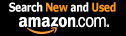CTK Exchange Front Page Movie shortcuts Personal info Awards Reciprocal links Terms of use Privacy Policy Cut The Knot! MSET99 Talk Games & Puzzles Arithmetic/Algebra Geometry Probability Eye Opener Analog Gadgets Inventor's Paradox Did you know?... Proofs Math as Language Things Impossible My Logo Math Poll Other Math sit's Guest book News sit's Recommend this site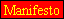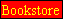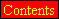|Store|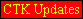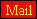CTK Exchange

 Subject: "Cassini's determination of distance to Sun" Previous Topic | Next Topic
 ConferencesThe CTK ExchangeHigh schoolTopic #325Printer-friendly copyEmail this topic to a friend Reading Topic #325
alexb
Charter Member
1592 posts
Jul-13-05, 11:27 AM (EST)"Cassini's determination of distance to Sun"

 In 1672 a chap named Cassini found the distance from the earth to the sun. He used a scheme which required that he send a friend to South America while he stayed in Paris, and both of them looked at Mars on the same day just as it was rising (as I understand it). The details are given at https://www.astronomyforbeginners.com/astronomy/howknow.php#distsun Each observed the star that was in the background behind Mars. Then they got together again, and by knowing the identity of the two stars, were able to figure the angle L (in the attached diagram). And from L and the radius of the Earth, they could find the distance to Mars (from R/D=sin(L/2)). Then from Kepler's third law they could find the distance to the sun. The trouble is, I am unable to see how to compute L from the information (their angular displacement in the sky, presumably) on the two stars. Can you see how to do it? I suspect it's obvious, and it's just my blindsightnedness that keeps me from understanding. Monty

Subject     Author     Message Date     ID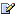Cassini's determination of distance to Sun alexb Jul-13-05 TOPRE: Cassini's determination of distance to Sun alexb Jul-14-05 1RE: Cassini's determination of distance to Sun mr_homm Jul-15-05 2RE: Cassini's determination of distance to Sun Monty PhisterJul-15-05 3RE: Cassini's determination of distance to Sun alexb Jul-15-05 4RE: Cassini's determination of distance to Sun mr_homm Jul-15-05 5RE: Cassini's determination of distance to Sun MontyJul-20-05 6RE: Cassini's determination of distance to Sun mr_homm Jul-21-05 8RE: Cassini's determination of distance to Sun AFBJul-20-05 7RE: Cassini's determination of distance to Sun mr_homm Jul-21-05 9

 Conferences | Forums | Topics | Previous Topic | Next Topic
alexb
Charter Member
1592 posts
Jul-14-05, 07:02 PM (EST)1. "RE: Cassini's determination of distance to Sun"
In response to message #0

 The essential thing to remember is that at the night Cassini took measurements and determined the distance to Mars, Sun, Earth and Mars were collinear and Mars was farther from the Sun, so that, at that time,dist(Sun, Mars) = dist(Sun, Earth) + dist(Earth, Mars)1.542 AU = 1 AU + d,where d is the measured dist(Earth, Mars). ThusAU = d/.542How did they find d?They assumed to make measurements on the opposite locations on earth. The two points of measurements and Mars formed an isosceles triangle whose base was known diameter of Earth. What they used their measurements for was to determined the angle at the apex (Mars). (The formula actually uses tan() and sin()).How did the find the angle?As a guess, the star in question may have been assumed to be infinitely far, so that both lines from the points of observation to the star may be thought as being perpendicular to the diameter of Earth between the points of measurement. (Far as I understand, their result was of by 7%. Perhaps, because of an assumption like that.) The two fellows measured the angles between the lines to Mars and to the star. If you draw a diagram you'll see that the angle we are after is the sum of the two measured angles.mr_homm
Member since May-22-05
Jul-15-05, 11:08 AM (EST)2. "RE: Cassini's determination of distance to Sun"
In response to message #1Monty Phisterguest
Jul-15-05, 01:25 PM (EST)

3. "RE: Cassini's determination of distance to Sun"
In response to message #2

 Regarding "how did they find d", and "how did they find the angle": The two cities from which the observations were taken were in fact not on a common diameter, so there is no "diameter of Earth between the points of measurement." The observations were taken AT THE SAME TIME, so there wouldn't be an isosceles triangle whose base is the diameter of the earth. After the results of the observations were joined, Cassini could measure the angle between the two stars, from a common point. But how is that angle related to d?alexb
Charter Member
1592 posts
Jul-15-05, 01:28 PM (EST)4. "RE: Cassini's determination of distance to Sun"
In response to message #3

 > The two cities from which the observations were taken >were in fact not on a common diameter, so there is no >"diameter of Earth between the points of measurement." The >observations were taken AT THE SAME TIME, so there wouldn't >be an isosceles triangle whose base is the diameter of the >earth. mr_homm explained this point: so there were two points with a known between them. The points were not diametrically opposite.>After the results of the observations were joined, >Cassini could measure the angle between the two stars, from >a common point. They did not measure the angle between two stars but between a star and Mars.>But how is that angle related to d? Please see the previous message. The angle related to d was the sum of two they measured.mr_homm
Member since May-22-05
Jul-15-05, 02:25 PM (EST)5. "RE: Cassini's determination of distance to Sun"
In response to message #3

 >Regarding "how did they find d", and "how did they find the >angle": > The two cities from which the observations were taken >were in fact not on a common diameter, so there is no >"diameter of Earth between the points of measurement." The >observations were taken AT THE SAME TIME, so there wouldn't >be an isosceles triangle whose base is the diameter of the >earth. This is correct. Further, if the triangle is NOT isoceles, there is no direct relationship between the angle and the distance d to Mars. For the case of an isoceles triangle, you only need to know the base (distance between measurement points on Earth) and the angle at the apex (Mars), because for an isoceles triangle, knowing one angle will determine the other two for you.If you do not have an isoceles triangle the situation is more complicated, but can still be handled. It is easier to use rectangular coordinates to do the math, so that's how I will proceed:Let's call Paris "P",the other observation point "Q" and Mars "M". The known latitude and longitude of P and the radius of the earth describe the position of P relative to the center of the Earth in spherical coordinates r = earth radius, theta = 90 degrees - latitude, phi = longitude. Convert these to x, y, z coordinates by z=r*cos(theta), x = r*sin(theta)*cos(phi), y = r*sin(theta)*sin(phi). Do the same for Q. Now you have the coordinates of both points, so subtracting one set from the other gives the vector PQ. The length of PQ is the straight line distance between the two observation points. Now divide the vector PQ by this length to make it into a vector with length = 1. The observation of M in the sky from P gives the celestial coordinates of M, which are declination ( same as latitude) and right ascension (same as longitude, expressed in units of hours, where 24 hours = 360 degrees). We of course don't know the distance coordinate "r" for M yet, as that's what we're trying to find. Assume r=1 for M as seen from P, and convert to rectantular coordinates as before.Now you have a vectors of length 1 from P to Q and from P to M. Take the dot product of these two vectors (product of x components + product of y comoponents + product of z components). This is the cosine of the angle between the two vectors, so applying inverse cosine gives the angle at P in the triangle QPM. Since Cassini can measure the angle at M by looking at the two reference stars, he now has two of the three angles. Since the angle sum up to 180 degrees, this gives him the third angle.Knowing all three angles and the straight line distance PQ, he can use the law of sines to find the distances QM and QP. Notice that this doesn't give the distance from Mars to the center of the Earth, but instead from Mars to Paris. Of course, thid difference is negligible in comparison to the scale of the solar system.I think this is what you were looking for, right? I have deliberately given a lot of detail about the calculation in order to show how it would actually be carried out. The short answer to your question would have been: Knowing the positions of P and Q, Cassini knew the length and orientation of the line joining them. From this and the known directions of the sight lines to Mars, he could compute the angles at the two nearby corners of the triangle, and his observations of the background stars gave him the angle at Mars. Then he had enough information to apply the law of sines. > After the results of the observations were joined, >Cassini could measure the angle between the two stars, from >a common point. But how is that angle related to d? Now that you have the answer to this, I should say that the situation you find yourself in is a common one. People who are trying to explain something (for example astronomy) at an introductory level usually try to hide the messy details so that the central idea can be clearly seen. However, the details are often necessary, and so the clarity is only apparant. Inevitably someone (you in this case) comes along and takes a deeper look at the presentation and asks a question that can't be answered from the oversimplified explanation, and all these messy details come rushing right back into the discussion. This is a good thing, in my opinion, because actual understanding often means resisting the oversimplified presentation and demanding the details.--Stuart AndersonMontyguest
Jul-20-05, 06:10 PM (EST)

6. "RE: Cassini's determination of distance to Sun"
In response to message #5

 Right. That's EXACTLY what I was looking for, and I think I finally understand. I have a math newsletter https://www.gnarlymath.com/news/gnews1_1.html and am working on an "astronomy" issue. I was hoping the math would be simple enough that I could include it in the newsletter, but it won't do. So I'll be "leaving the messy details" (or at least many of them) out. I hope to do a little better than was done on the page referenced in my first note, though.While I was puzzling over this, another idea came to mind. Would Cassini have been able to predict the time when Mars was in such a position that a line from Mars to the earth's center was perpendicularto the earth's diameter? Putting it another way: consider a plane passing through P, the center of the Earth, and Mars. The plane will rotate as Mars and the Earth move. At some point, the line from Mars will be perpendicular. If he then measured the angle from Paris to Mars, could he compute the Earth-Mars distance from that angle and the latitude/longitude of Paris? In other words, could he have found the Mars distance without sending his buddy off to the ends of the Earth? One needs two positions to locate Mars. But could one of them be the Earth's center instead of Cayenne?mr_homm
Member since May-22-05
Jul-21-05, 09:53 AM (EST)8. "RE: Cassini's determination of distance to Sun"
In response to message #6AFBguest
Jul-20-05, 06:36 PM (EST)

7. "RE: Cassini's determination of distance to Sun"
In response to message #5

 Hi, I have been reading this thread with interest, as Astronomy for Beginners is my site. Thanks for the clarification Stuart (and for the derivation), I never really thought about it that much detail and I agree my article was oversimplified. I changed the text in the main article and removed the offending equations, and put a link to a new page (https://www.astronomyforbeginners.com/astronomy/mars.php) with the mathematics in more detail, so if people want to know more detail its available.ThanksGavinmr_homm
Member since May-22-05
Jul-21-05, 10:12 AM (EST)9. "RE: Cassini's determination of distance to Sun"
In response to message #7

 You're welcome, Gavin. I'm glad you found the material useful.By the way, sorry if it'seemed I was criticising your site with the word "oversimplified." I really just meant that it is necessary to simplify the initial explanation to make it clear, but later if you want to go deeper, that explanation won't do any more. So from that point of view, the explanation was oversimplified, but this kind of oversimplification really is often the best way to introduce a concept.I just took another look at your website, and I really like what you've done with the explanation I gave. Keeping it as simple as possible on the front page, then having a link to more details is (I think) the best and clearest way to present it. In fact, it is clearer now than the way I said it, because you have explained things that a general audience might not know, such as unit vectors, law of sines, and using the vector "dot" product to find the cosine of an angle. I just assumed those things in my posting, because I knew I was talking to people who would know them. It's good to see a website whose owner really thinks about his audience and what they might need to know. Very nicely done!--Stuart Anderson

 Conferences | Forums | Topics | Previous Topic | Next Topic
 Select another forum or conference Lobby The CTK Exchange (Conference)   |--Early math (Public)   |--Middle school (Public)   |--High school (Public)   |--College math (Public)   |--This and that (Public)   |--Guest book (Public) Educational Press (Conference)   |--No Child Left Behind (Public)   |--Math Wars (Public)   |--Mathematics and general education (Public)You may be curious to have a look at the old CTK Exchange archive.